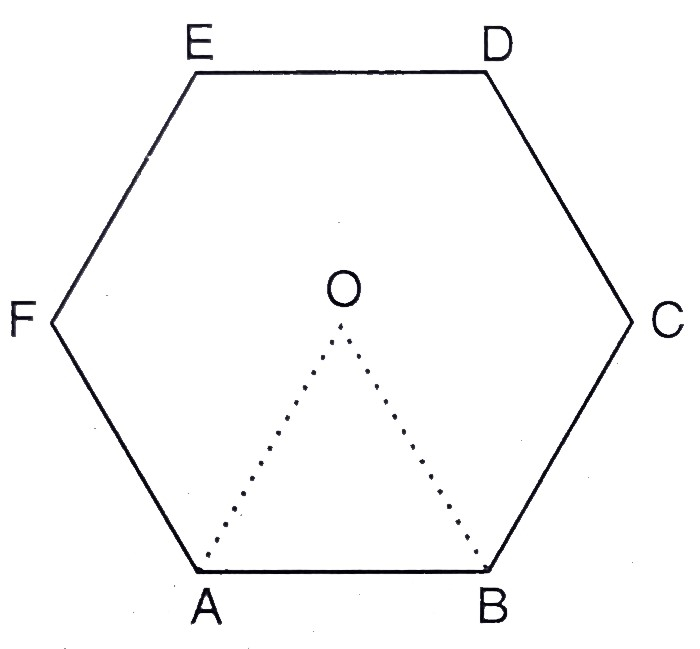"
">

# $A B C D E F$ is a regular hexagon with centre $O$. If the area of triangle $O A B$ is 9 $\mathrm{cm}^{2}$, find the area of the hexagon."

Given:

$A B C D E F$ is a regular hexagon with centre $O$.

The area of triangle $O A B$ is 9 $\mathrm{cm}^{2}$.

To do:

We have to find the area of the hexagon.

Solution:

We get six equal equilateral triangles by joining the vertices of the hexagon with $O$.

Area of the equilateral triangle $OAB = 9\ cm^2$.

This implies,

Area of hexagon $= 9 \times 6\ cm^2$

$= 54\ cm^2$

The area of the hexagon is $54 \mathrm{~cm}^{2}$.

Updated on: 10-Oct-2022

69 Views